# Chapter 10 Example

## Catalyst Decay in a Packed Bed

The reaction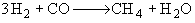is carried out with excess H2 in a long packed-bed reactor containing a Ni–Mo–Cu catalyst. The reaction is first order in CO. The rate of catalyst decay is first order in CO and first order in the catalytic activity. The exiting carbon monoxide concentration was monitored as a function of time in two separate experiments, one at a high volumetric flow rate and one at a low volumetric flow rate.

For a space time of 1.0 h:

 t (h) 1 4 8 12 16 20 24 CO (mol/dm3) ´ 102 0.05 0.11 0.3 0.58 0.82 0.93 0.98

For a space time of 100 h:

 t (h) 10 500 1000 1050 1090 1095 1100 1110 1120 1200 CO (mol/dm3) ´ 102 0 0 0 0 0.047 0.18 0.5 0.95 0.99 1

Expectations

Initially the reactor is filled with inert gas and at time t = 0 the reactant gas mixture is fed to the reactor. At the start, the reactant gas sees only fresh catalyst and methane is produced. As time goes on the catalyst at front of the reactor becomes spent as a result of being poisoned by CO and the entering reactant gas must travel further down the reactor to reach fresh catalyst.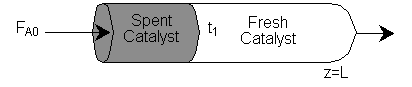As time continues, the activity front moves on down the reactor and eventually breaks through the end of the reactor at time called the break through time tb. The figure below shows the activity profile down the reactor as a function of time. The activity profile shown is relatively steep, but the steepness will be a function of the reaction rate and the flow rate.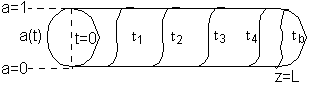Activity Profile as a function of time.

If one were to look at the exit, (z = L) the conversion of CO, it might look something like

either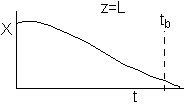or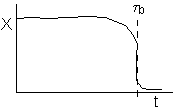(a)                                                  (b)

(a) or (b) depending on the rate of reaction and the Damköhler number. We note that same phenomena occurs in similar systems such as the leaching of uranium, the acidization of petroleum wells, and in chromatographic reactors.

#### Analysis

Packed bed reactor with catalyst decay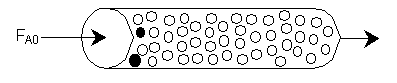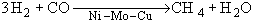Assumptions

(1)     Neglect volume change because of XS H2 (yA0 << 1  \  e @ 0)

(2)     Neglect pressure drop

(3)     Neglect axial dispersion

(4)     Neglect CO, consumed to Poison sites

#### A.  The Algorithm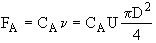Mole Balance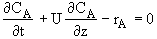Rate Law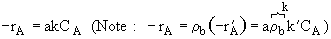Decay Law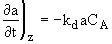Stoichiometry                      Gas phase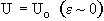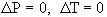Combine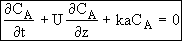(1)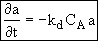(2)

Initially, there is an inert gas in the reactor and at time t = 0 we will begin feeding the fresh reactant gas.

 IC t = 0 CA = 0 z > 0 BC z > Ut a = ao (ao = 1) (3) z = 0 t > 0 CA = CA0

#### B.  Putting the Equations in Dimensionless Form

Let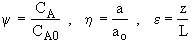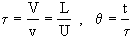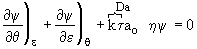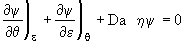Da = Damköhler number which gives the shape of decay front =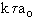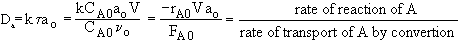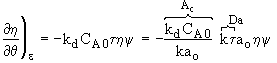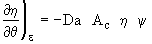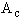= Activity Number which is related to the velocity of decay the front =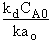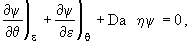(4)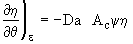(5)

 IC θ = 0 ε > 0 ψ = 0 BC ε ≥ 0 η = 1 θ ≥ 0 ε = 0 ψ = 1

Combining Equation (1) and (2)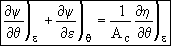(6)

#### C.  Applying the Method Of Characteristics

Recall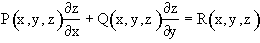with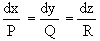Let’s see what functional forms the transformation suggests for the above equation describing reaction and decay in a packed bed.

Integrating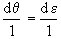the characteristic is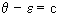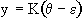Since K is an arbitrary constant we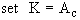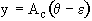(Note: at ε = 0 and θ = 0, ∴ y = 0 and ψ = 1)                                                              (7)

#### D.  Using the characteristic y to obtain the exact differential Function dF = ηdε+ ψdy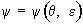Then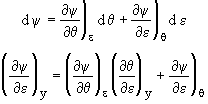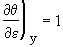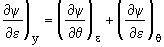(8)

From Eqns (6) and (8)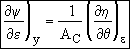(9)

Combine Eqns (5) and (9)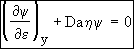(10)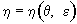Then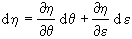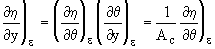(11)

Combining Eqns (11) and (9)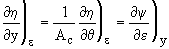Exact Differential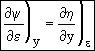(12)

The above equation is an exact differential. Therefore a function F exists such that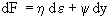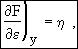(13)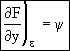(14)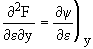(15)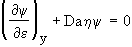(10)

Substituting Eqns (13), (14) and (15) into Eqn (10)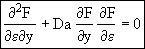(16)

#### E.  Solving the Hyperbolic PDE

This is a Hyperbolic PDE and there are known transforms to solve hyperbolic PDEs

Let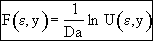(17)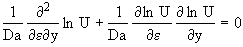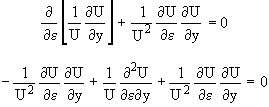Then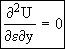(18)

The most general solution is(19)

Recalling Eqn (14)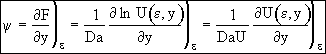(20)

at ε = 0   ψ = 1, Eqn. (20) becomes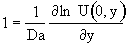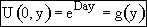(21)

Recalling Eqn (13)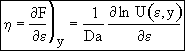(22)

at y = 0   η = 1, Eqn (21) becomes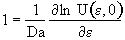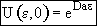(23)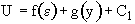(19)

Substituting Eqns (21) and (23) into Eqn. (19)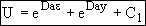(24)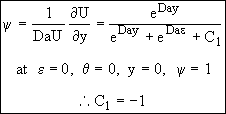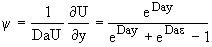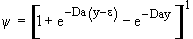(25)

#### F.  Putting everything back in terms of Da and Ac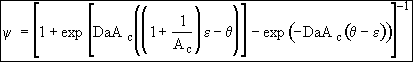(26)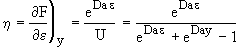(27)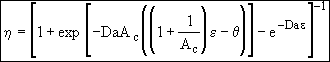(28)

#### G.  Evaluating the Parameters form Experimental Data

At exit,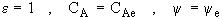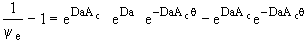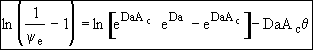(29)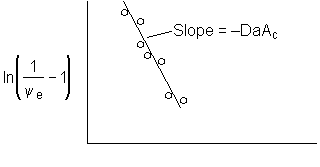Figure 1. Dimensionless exit concentration function versus q

We have used data the concentration ratio, (ψe = CAe/CA0) at the exit, z=L, to evaluate the product DaAc. We will now evaluate the activity number, Ac, from the movement of the reaction front and the time it takes to break through at the end of the reactor, z=L.

#### H.  Movement of the Reaction Front

Look at the exponential terms in Eqn (26) that are multiplied by DaAc. The arguments are zero when θ = ε and when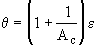. These relationships give the movement of two fronts. This front gives the dimensionless position of fresh gas interfaceThis front gives the dimensionless position of the decay activity interface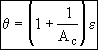Now that we have the product DaAc from the slope of Figure 1. At breakthrough of the activity front at the end of the reactor ε = 1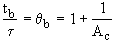The breakthrough time is used to calculate the activity number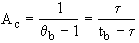#### I.  Position of Reaction (Decay) Front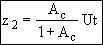High DaLow Da

Figure 2. Profile of y and h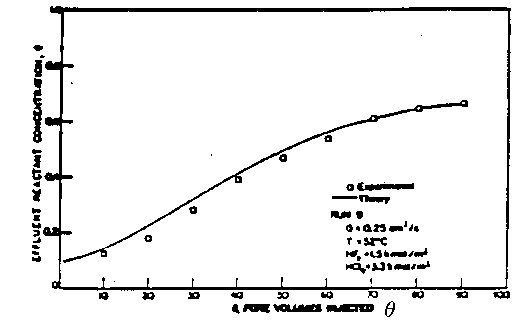Figure 3. Comparison of Theory and Experiment

From Lund and Fogler Chem. Engrg. Sci. 31 p381 (1976).

Back to Chapter 10